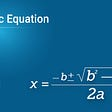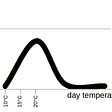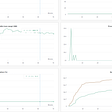# QC — Quantum Fourier Transform

Quantum Fourier Transform (QFT) is a critical part of Shor’s Algorithm and many other quantum algorithms. Its classical cousin is the Fast Fourier Transform. But there are some significant differences between them. In this article, we will take a look at QFT. But if you miss the first part of the Shor’s algorithm series, here is the link for your reference.

# Fast Fourier Transform FFT

FFT Properties

For engineerings and mathematician, FFT is no stranger. We use FFT to transform a signal in the time domain f(t) to the frequency domain ℱ(ω), or vice versa. One possible application is noise removal. We convert a signal to the frequency domain and remove the high-frequency noise. Then we convert the signal back to the time domain. In quantum computing, it is used for different purposes because of the limitation in measuring qubits.

If we have a sinusoidal wave with a 1Hz frequency, the corresponding frequency domain is a delta function with value equals 1.

FFT is symmetrical. If we have a delta function in the time domain, the FFT function becomes a sinusoidal function.

If it is a series of delta functions, the transformed function is a series of delta functions also.

If a function has a period r in the time domain, the transformed function has a period of 1/r in the frequency domain.

FFT Definition

The Fourier Transformation is mathematically defined as:

So it becomes natural that we can compute the Fourier Transformation with some phase shifting gates in quantum computing.

# Quantum Fourier Transform (QFT)

The quantum Fourier transform is defined as:

As shown before, a delta function should be transformed to a sinusoidal function.

i.e., |10⟩ will transform into the superposition below:

As we know, Fourier Transform is reversible. Quantum Fourier Transform (QFT) is reversible also. To prove that QFT is implementable, we need to prove the transformation is unitary. i.e. the Fourier transform F fulfills the following condition:

Given F is defined as:

We can prove below that the operation is unitary. Multiple it with its conjugate transpose equals I.

Let’s reformulate the QFT as a linear operator:

where

All the power polynomial of ω lie on the unit circle below:

For N=4,

For example, to transform the vector:

We simply perform

Let ’s repeat the calculation with different inputs, and here are the results.

In Fourier Transform, we develop a faster version called Fast Fourier Transform to compute the transformation iteratively. Now, we are going to do the same thing on the quantum version.

First, we will introduce some notation first.

For j=2, we can write it in a binary form 10 with j1=1 and j2=0.

The binary form 0.10 (0. j1 j2) equals to 0.5 in decimal.

It can be proven here that we can perform the QFT iteratively as:

A controlled-R quantum gate applies a relative phase change to |1>. The matrix form of this operator is:

So the QFT can be implemented with a series of the controlled-R gate as below:

Consider multiple R2 with j2.

Therefore, the result for qubit j1 before the switching at the end is.

Let’s take out the annotation for better clarity.

Here is an example of the circuit:

Difference between QFT and FFT

The general formula for QFT is:

In quantum computing, loading the coefficient (αj) for the computation bases is not very simple. Also, there is no effective way to retrieve the coefficient (αk) of the computation bases after the transformation. The measurement returns the computation basis, not the coefficient!

But don’t be disappointed. As a standalone algorithm, it may not be very powerful. Its potential will be realized later when combining with other algorithms. For example, it can measure the period of a function that is needed to crack the RSA algorithm.

# Next

Next, we need to understand an abstract concept called phase estimation before we can put everything together to solve the prime factorization.

--

--

--

## More from Jonathan Hui

Deep Learning

Love podcasts or audiobooks? Learn on the go with our new app.

## How to become a good problem solver?## C Program to find the roots of a Quadratic Equation## What is the Difference Between Speed and Velocity? — The Prodigious## Monopoly Math## Monty Hall Problem will drive you crazy## Statistics & Probability: Fundamentals 2Deep Learning

## (A piece of) Machine Learning revolution in practice## WandB: Alternative to TensorBoard more intresting thing about.## My first in-person conference experience! CVPR 2022## What I Learned While Implementing Computer Vision Papers